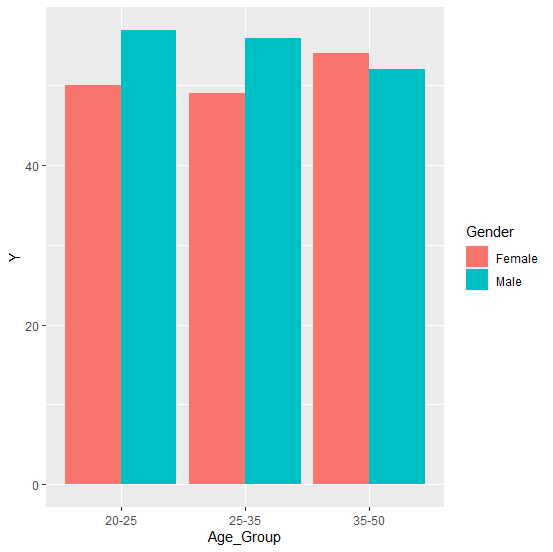# How to create bar chart based on two groups in an R data frame?

R ProgrammingServer Side ProgrammingProgramming

To create a bar chart based on two groups, we can use geom_bar function of ggplot2 package with position argument that defines the position of the groups. For example, if we have a data frame called df that contains two categorical variable x1 and x2 and the one response variable y then the bar chart can be created by using the below command −

ggplot(df,aes(x1,y,fill=x2))+geom_bar(position=position_dodge(),stat="identity")

## Example

Live Demo

Consider the below data frame &minus

Gender<−sample(c("Male","Female"),20,replace=TRUE)
Age_Group<−sample(c("20-25","25-35","35-50"),20,replace=TRUE)
Y<−rpois(20,50)
df<−data.frame(Gender,Age_Group,Y)
df

## Output

  Gender Age_Group Y
1 Male    20−25     57
2 Female  35−50     49
3 Male    20−25     57
4 Male    20−25     48
5 Male    35−50     52
6 Male    25−35     56
7 Male    35−50     49
8 Male    25−35     55
9 Male    20−25     45
10 Male   20−25     52
11 Male   20−25     55
12 Female 35−50     48
13 Male   20−25     47
14 Male   20−25     44
15 Male   35−50     47
16 Male   35−50     44
17 Female 25−35     49
18 Male   35−50    45
19 Female 35−50     54
20 Female 20−25     50

Loading ggplot2 package and creating the bar chart based on x1 and x2 −

## Example

library(ggplot2)
ggplot(df,aes(Age_Group,Y,fill=Gender))+geom_bar(position=position_dodge(),stat="identity")

## Output